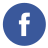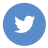### Client-side validation of credit cards

javascript

To perform client-side validation of credit card numbers in JavaScript, you can use the Luhn algorithm. The Luhn algorithm is a checksum formula that is used to validate a variety of identification numbers, including credit card numbers.

Here’s an example of how to implement the Luhn algorithm to validate a credit card number in JavaScript:

``````function validateCreditCardNumber(cardNumber) {
// Remove any spaces or dashes from the card number
cardNumber = cardNumber.replace(/\s+/g, '').replace(/-/g, '');

// Check that the card number contains only digits
if (!/^\d+\$/.test(cardNumber)) {
return false;
}

// Check that the card number is a valid length
if (cardNumber.length < 13 || cardNumber.length > 19) {
return false;
}

// Perform the Luhn algorithm
var sum = 0;
var shouldDouble = false;
for (var i = cardNumber.length - 1; i >= 0; i--) {
var digit = parseInt(cardNumber.charAt(i));

if (shouldDouble) {
digit *= 2;
if (digit > 9) {
digit -= 9;
}
}

sum += digit;
shouldDouble = !shouldDouble;
}

return (sum % 10) == 0;
}``````

In this example, we define a validateCreditCardNumber function that takes a credit card number as input and returns true if the number is valid and false otherwise.

The function first removes any spaces or dashes from the card number using regular expressions. It then checks that the card number contains only digits and is a valid length (between 13 and 19 digits).

Finally, the function performs the Luhn algorithm by iterating over the digits of the card number from right to left. For each digit, it doubles the value if it should be doubled (according to the Luhn algorithm) and adds it to the sum. It then toggles the shouldDouble flag to determine whether the next digit should be doubled.

After iterating over all the digits, the function returns true if the sum is divisible by 10 (indicating that the card number is valid) and false otherwise.

You can call this function on the client side to validate credit card numbers entered by users before submitting them to a server for processing.